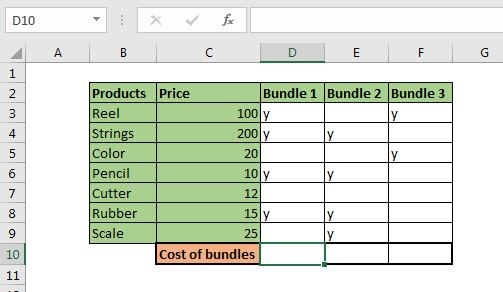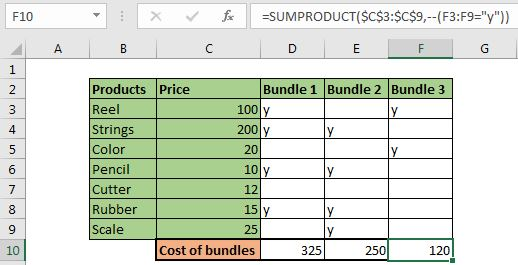# Excel Calculator For Bundle Pricing using SUMPRODUCT

Let's say you have a list of products with their price in an Excel table. Now you need to create bundles that may contain different products from available items. Now, in the end, you would want to calculate the total price of each bundle. For one time you may do it manually, but if this your regular task then it is better to automate this task with some elegant formulas. And this is what this article for. We will learn how to calculate the pricing of bundles or groups of items using one formula.

Generic formula:

 =SUMPRODUCT(price_range,--(check_range="y"))

price_range: It is the range that contains the price of the products.

check_range: It is the range in which we want to put our check. If a product is part of the bundle, we put y in the cross-section of bundle and product.

"y"= This the check we are putting to include a product in the bundle.

Let's have an example to clear the concept.

Example: Create Formula for Pricing of Bundles in Excel.We take the same scenario that we discussed in the beginning. We have prepared the table in range B2:F9. Let's identify the variables we need.

Price range: The price range is C2:C9. Since it is fixed we can either named range or use the absolute reference of it. In this example, I will use absolute reference \$C\$2:\$C\$9.

Check_range: These are the range that contains checks (the bundle column). They are D3:D9, E3:E9, and F3:f9.

Let's put these values in the generic formula.

Write this formula in D10 to calculate the bundle price.

 =SUMPRODUCT(\$C\$3:\$C\$9,--(D3:D9="y"))

Hit enter. You have the bundle cost of bundle 1 calculated in cell D10. Copy this formula to adjacent cells to calculate the price of all the bundles.How does it work?

The formula works inside out. So first --(D3:D9="y") is solved.

This returns an array of 1, and 0 as. 1 for every y and 0 anything else in range D3:D9.

 {1;1;0;1;0;1;0}

Next, the \$C\$3:\$C\$9 is converted into an array that contains the price of each item/product.

 {100;200;20;10;12;15;25}

Now the SUMPRODUCT function has this in it.

 =SUMPRODUCT({100;200;20;10;12;15;25},{1;1;0;1;0;1;0})

Now as the SUMPRODUCT function does, it multiplies each value in one array to the same indexed array in other array and finally sums up those values. It means that each price that matches to 0 in other array are turned into 0. {100;200;0;10;0;15;0}. Now, this array is summed. This gives us 325 for bundle 1. The same is done for all bundles.

Alternative formula:

The alternative formula is, of course, the SUMIF and SUMIFS function.

 =SUMIF(D3:D9,"y",\$C\$3:\$C\$9)

and

 =SUMIFS(\$C\$3:\$C\$9,D3:D9,"y")

These are the classic answers but the SUMPRODUCT formula is faster and fancy too.

So yeah guys, this how you can calculate the price of the bundle in excel easily. I hope it was explanatory enough. If I missed any point or you have any doubts regarding this article or any other excel related doubt, ask it in the comments section below.

Related Articles:

Count total matches in two ranges in Excel | Learn how to count total matches in two ranges using SUMPROUDCT function.

SUMIFS using AND-OR logic | The SUMIFS can be used with OR logic too. The defualt logic SUMIFS uses is AND logic.

SUMPRODUCT with IF logic | Learn how to use SUMPRODUCT with IF logic without using IF function in the formula.

Related Article:

50 Excel Shortcuts to Increase Your Productivity | Get faster at your task. These 50 shortcuts will make you work even faster on Excel.

The VLOOKUP Function in Excel | This is one of the most used and popular functions of excel that is used to lookup value from different ranges and sheets.

COUNTIF in Excel 2016 | Count values with conditions using this amazing function. You don't need filter your data to count specific value. Countif function is essential to prepare your dashboard.

How to Use SUMIF Function in Excel | This is another dashboard essential function. This helps you sum up values on specific conditions.

Terms and Conditions of use

The applications/code on this site are distributed as is and without warranties or liability. In no event shall the owner of the copyrights, or the authors of the applications/code be liable for any loss of profit, any problems or any damage resulting from the use or evaluation of the applications/code.